# Pontryagin square

A cohomology operation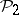of type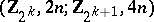, i.e. a functorial mapping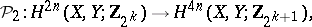defined for any pair of topological spaces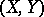and such that for any continuous mapping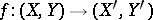the equality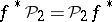(naturality) holds.

Pontryagin squares have the following properties:

1), whereis the natural imbedding.

2)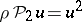and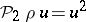, where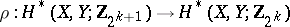is the quotient homomorphism modulo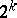.

3)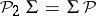, whereis the suspension mapping and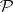is the Postnikov square (in other words, the cohomology suspension ofis). If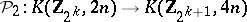and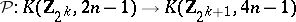are the representing mappings, then.

The properties 1), 2) uniquely characterize the Pontryagin square and thus can be taken as an axiomatic definition of it. Constructively the Pontryagin square is defined by the formula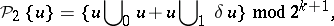where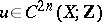is a cocycle modulo(for the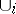-products see Steenrod square).

There exists (see , ) a generalization of the Pontryagin square to the case whenis an arbitrary odd prime number. This generalization is a cohomology operation of typeand is called the-th Pontryagin power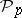. The operationis uniquely defined by the following formulas: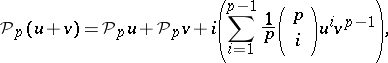whereis the natural imbedding; and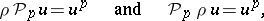whereis the quotient homomorphism modulogeneralizing the corresponding formulas for. The analogue of formula 3) forhas the form, which means that the cohomology suspension offoris zero. Forthe equalityholds, the multiplication may be taken both as outer (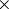-multiplication) or inner (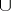-multiplication). For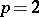the corresponding equality is valid only up to summands of order 2.

In the most general way the Pontryagin square is defined for cohomology with coefficients in an arbitrary finitely-generated Abelian group(see , ). In final form this generalization is as follows (see ). The Pontryagin square is a ring homomorphism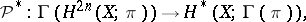whereis a functor which associates a ring with divided powers to an Abelian group. For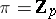, the-th component of this homomorphism coincides with the-th Pontryagin power(forwith the Pontryagin square).